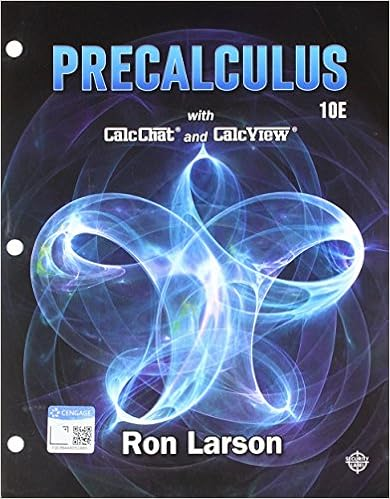# Problems 1 a particle has such a curvilinear motion

• Notes
• 8
• 100% (3) 3 out of 3 people found this document helpful

This preview shows page 6 - 8 out of 8 pages.

##### We have textbook solutions for you!
The document you are viewing contains questions related to this textbook.The document you are viewing contains questions related to this textbook.
Chapter 10 / Exercise 98
Precalculus
Hostetler/LarsonExpert Verified
PROBLEMS: 1.A particle has such a curvilinear motion that its x-coordinate is defined by x= 5t3– 105t where x is in inchesand t in seconds. When t = 2 sec., the total acceleration is 75 in/sec2. If the y-component of acceleration isconstant and the particle starts from rest at the origin when t = 0. Determine (a) the y-component of theacceleration when t = 2 sec., (b) the vertical component of the velocity when t = 4 sec., and (c) the totalvelocity when t = 4 sec.2.At any instant the horizontal position of the weather balloon in the figure is defined by x = (8t) ft, where t isin seconds. If the equation of the path is y = x2/ 10, determine (a) the distance of the balloon from the stationat A when t = 2 sec., (b) the magnitude of the velocity when t = 2 sec., and (c) the magnitude of theacceleration when t = 2 sec.=vx2+vy2a=dvdt=axi+ayja=ax2+ay2Ay = x²/10xyax= ´vxxay= ´vy= ´y
3.A rocket was launched vertically, when the rocket reaches altitude h1 it begins to travel along the parabolicpath bx = (y – h1)2. If the component of velocity in the vertical direction is constant at vy= vo, determine themagnitudes of the rocket’s velocity and acceleration when it reaches altitude h2. Assuming the values are,v=vx2+vy2a=dvdt=axi+ayja=ax2+ay2Ay = x²/10xyax= ´vxxay= ´vy= ´y
##### We have textbook solutions for you!
The document you are viewing contains questions related to this textbook.The document you are viewing contains questions related to this textbook.
Chapter 10 / Exercise 98
Precalculus
Hostetler/LarsonExpert Verified
MECHANICS - DYNAMICS MOTION OF A PROJECTILEThe free flight of a projectile is often studied in terms of its rectangularcomponents, since the projectile’s acceleration always acts in the verticaldirection. To illustrate the kinematic analysis, consider a projectilelaunched at point (xo, yo), as shown in Figure 10. The path is defined in thex-y plane such that the initial velocity is vo, having components (vo)xand(vo)y. When air resistance is neglected, the only force acting on theprojectile is its weight, which causes the projectile to have a constantdownward acceleration of approximately a = g = 9.81 m/s2or 32.3 ft/s2a = - gjO(v )xyyyxxooy(v )voooxvvvxyrFig. 10.
MECHANICS - DYNAMICS PROBLEMS:
•••